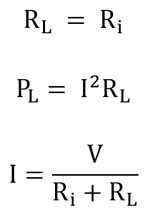# Maximum Power Transfer Theorem

In electrical components, internal resistance occurs due to the resistance of the electrolyte. When a load is connected to the supply, the current flows through the internal resistance of the supply, causing the drop in voltage. The transference of maximum power from a supply to a load is required in electronic circuits, which is explained using the circuit diagram below.

FIGURE

According to this theorem, maximum power is developed in a load when the load resistance (RL) is equal to the internal resistance (Ri) of the supply.When the load resistance and the supply resistance are equal, they are said to be matched.